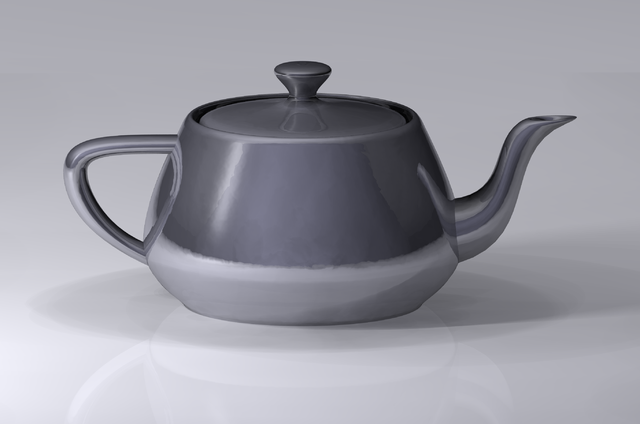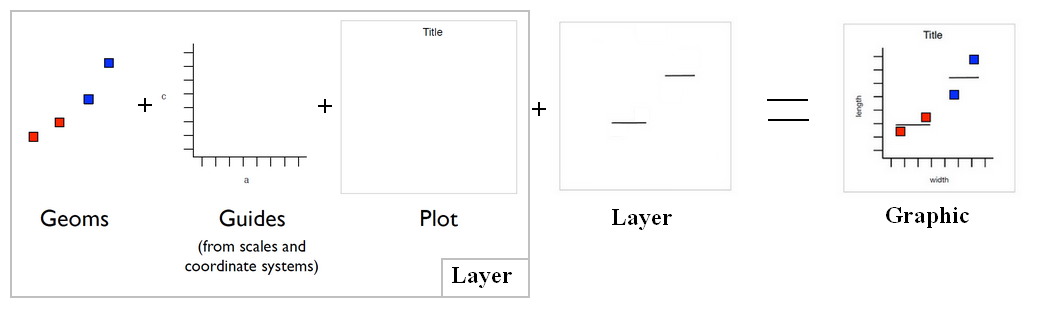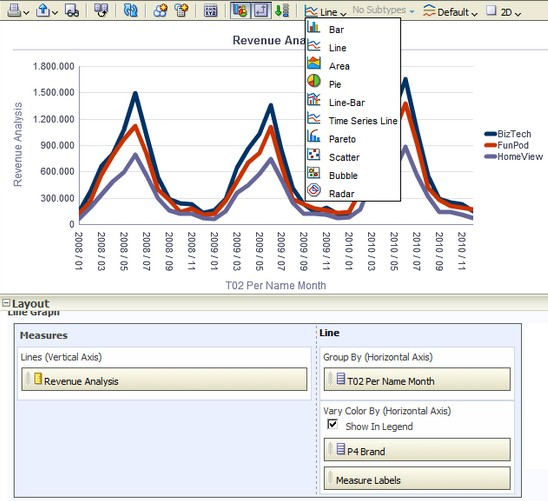# Data Visualization - Bar Chart

Bar graphs plots:

• associated with quantities (number of case, sum of)

whereas an histograms plots:

They show:

• quantities as bar lengths
• and categories as bars or groups of bars.

They show

The bars can be in a horizontal or vertical direction.

## Example

• competing product sales, same product sales over different time periods,
• same product sales over different markets.

## Subtype

• Vertical
• Horizontal
• Stacked Vertical
• Stacked Horizontal

## Styles

• Rectangle
• Triangle
• Cylinder
• Diamond
• Pattern Fill

Recommended PagesD3 - Bar Chart

in D3Data Visualisation - Histogram (Frequency distribution)

A histogram is a type of graph generally used to visualize a distribution An histogram is also known as a frequency distribution. Histograms can reveal information not captured by summary statistics...Data Visualization - ( Chart | Graph | Graphic)

A chart, also called a graph (in mathematics for instance), is a graphical representation of data, in which the data is represented by symbols, such as: bars in a bar chart, lines in a line chart,...Data Visualization - Visual (or Mark)

A visual is an object created from visual primitive that gets the value of its properties from the data. See also: Visual Encoding Bar (ie rectangle) Point (ie Circle or Square) Line Area...Data Visualization Foundation

The Utah Teapot. Data visualization is the process of mapping quantitative data to visuals (shapes, color, position, etc) to create a graph made of geometric object. Information visualization: ...Ggplot - Bar Chart

in Ggplot
HTML - Bar Chart

in HTML and CSS. HTMLd3 2436698The story of the teapotOBIEE 10G/11g - (Chart|Graph) View

A graph view displays numeric information visually, which makes it easier to understand large quantities of data. For displaying a single data value, a gauge is often more effective than a graph. Graphs...